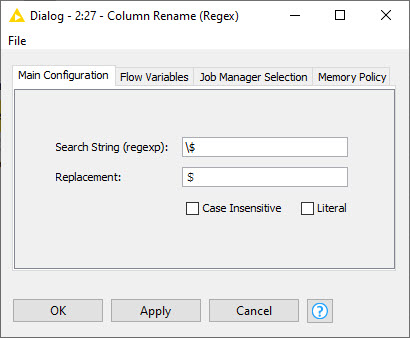# Math Formula - Using column headings with '\$'

I am trying to use Math Formula where my column headers have ‘\$’ signs in them.

However, because math formula uses ‘\$’ to identify the beginning and ending of a Column Name, I am getting an error.

For example,

Column 1 Name: Cost (\$)
Column 2 Name: Tax (\$)

Math formula: Cost (\$) + Tax (\$)
Knime Math Formula: \$Cost (\$)\$ + \$Tax (\$)\$

Hi @Rokank,

You can use the Column Expressions node instead.2 Likes

While this is a solution, is there a solution using the Math Formula node? I’m curious because I’ve noticed the math formula node calculates significantly faster than the column expressions node.

Actually it seems the node tries to escape the dollar sign inside the column name but for some reason it fails. So if you want to use the Math Formula, you have to rename columns and replace the dollar signs. I think the best approach to do that is using the Column Rename (Regex) node:This is an example expression after replacing dollar signs:
`\$Cost (＄)\$ + \$Tax (＄)\$`

You can copy and paste the alternative dollar sign in the expression to the “Replacement” option of the Column Rename (Regex) node.2 Likes

Hi!

Unfortunately not for now. But a workaround @armingrudd suggested seems pretty good to me.

Br,
Ivan

This topic was automatically closed 182 days after the last reply. New replies are no longer allowed.